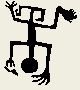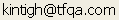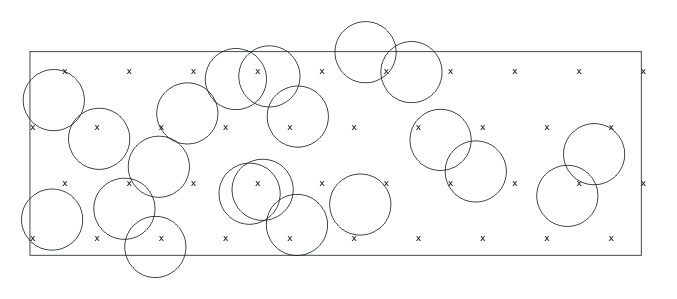Tools for Quantitative Archaeology    Email:HPPLOT: HP7475 Plot Utility

The primary use of HPPLOT is to create customized analysis graphics from a raw data file edited to include the plot commands. Included are commands to scale a plot, draw lines in a number of styles, fill areas in a number of ways, plot points with several different symbols, draw circles, rectangular windows, and pie charts, and put text of any size at any location on the plot.

HPPLOT provides a flexible user interface to a HP 7470, 7475 or compatible plotter. The output file can also be converted to Word Perfect graphics format and printed with Word Perfect using almost any printer (see "Incorporating plots in Word Perfect Documents" at the beginning of the documentation). However note that Word Perfect does not support all HPGL (Hewlett Packard Graphics Language) commands, in particular those for solid fill and pie charts.

The program can be used either in a batch mode, in which case an output file name is specified, or in an interactive mode. To run interactively specify "COM1" or "COM2" (for serial port 1 or 2 as appropriate) as the output file. In interactive mode, the plot will be done as the necessary commands are entered. In batch mode, to produce the plot copy the output file to the plotter, e.g.:

COPY SAMPLE.PLT COM1

At some time before the plot is actually made (either in interactive or batch mode), the serial port must be properly initialized for the plotter. This command will vary, depending upon how the plotter is configured. For a 9600 baud data rate with the plotter on serial port 1 type the DOS command:

MODE COM1:9600,,,,P

To start the HPPLOT program simply type:

HPPLOT

COMMAND DESCRIPTIONS

Commands remain active until they are changed. For example, once a height is specified, it is used for all text and symbol plotting. If a line style is specified to plot a line with the vector command and later one wished to plot a vector of individual symbols, the line style must be set to -2 or the symbols will be connected by the previous line's line style. Reference to the plotter language reference manual may clarify any problems. Note that the output plot file is a plain ASCII file that can be viewed (and changed) with a data editor.

Remember that although this program is flexible, it is "dumb". It will do what you tell it even if it makes no sense. However, it is "smarter" and a whole lot easier to use than the raw plotter commands.

All commands may be abbreviated to minimum number of distinguishing characters; in all cases a 2-character abbreviation will work. Parameters may be separated from the commands by any number of blanks commas or tab characters.

ANGLE <angle in degrees from horizontal> Subsequent text is plotted at this angle.

BOX <xmin> <ymin> <xmax> <ymax> Draws a rectangle with the corners specified and fills the rectangle with the active fill type.

CENTER <x1> <y1> <x2> <y2> <string> (center horizontal text between relative coordinates

CIRCLE <x> <y> <radius> Draws a circle centered at x,y and fills it with the active fill type

CLIP restrict drawing to window

DRAW <x1> <y1> <x2> <y2> Draw a line from point 1 to point 2

END End Plot

FILLTYPE Set the fill type for solid areas. 0=none, 1=hatched ///, 2= hatched \\\, 3= solid, 4=diagonal crosshatch, 5=vertical hatch, 6=horizontal hatch, 7=vertical & horizontal hatch. (Note: implement stippling by enabling line type for dots and dashes w/i the fill of a solid area.)

HEIGHT <Character Height in cm.>

HP <string> Pass remainder of line as HP7475 plotter command.

LABEL <x> <y> <string> place string at x,y measured absolutely in cm.

LINESTYLE <n> Set line style to n. ;-1 no line or dot
0 solid
1 dotted line
2 dashed line
3 long dashed line
4 dash dot
5 long dash, dash
6 long dash, dash, dash

NOCLIP stop restriction of drawing to window

PAPERSIZE A or B (8.5x11 or 11x17 respectively) Note resets window and scaling. In either case the x axis is the long axis.

PEN <n> Set Pen Number to n (1-6) to change colors or line width.

PIE <x> <y> <radius> <nseg> <v1> <v2> ... <vn> Creates a pie chart proportioned according to segments. Segment values are totaled and converted to proportions and need not add to 1 or 100.

SCALE <xmin> <xmax> <ymin> <ymax> Set scaled coordinates of lower left and upper right corner of window.

SYMBOL <c> or OFF or VARY Set current symbol to c. Off turns off symbol plot with vector. If VARY, the vector command reads a character to plot after each coordinate pair.

TEXT <x> <y> <string> Place string at x,y in relative coordinates.

VECTOR <n> <x1> <y1> ... <xn> <yn> (if SYMBOL ¬ VARY)

VECTOR <n> <x1> <y1> <c1> ... <xn> <yn> <cn> (if SYMBOL=VARY) Plot a vector of n points. In either case, SY is plotted at each vertex (unless off) and the points are connected or not according to linestyle. To plot symbols only use linestyle -2.

WINDOW <xmin> <ymin> <xmax> <ymax> Set plot window to absolute coordinates in cm. (If no WINDOW command is given a 2.5cm border is left all around.

SAMPLE INPUT

The commands used to produce the complex plot attached are explained here. The complete file (with the name HPPLOT.PLT) is provided on the program diskette. An abbreviated version is reproduced below.

The PA command sets the paper to 11x14 inch paper (B size). The WI command restricts the plot window (the area that is scaled) to a rectangle with its lower left corner 2 cm from the left edge of the paper and 1.5 cm above the bottom, and its upper right corner 32 cm from the left edge of the paper and 26.5 cm above the bottom. This rectangle is scaled (SC) such that the lower left corner of the box is (0, 0) in the analysis coordinates (meters from the grid origin) and the upper right corner is (40, 25). Thus the X data range 0-40 is displayed over the 30 cm horizontal of the plot and the Y data range 0-25 is displayed in the 25 cm of the vertical plot window. The resulting scale is 1 plot cm equals 1.33 world meters (or 1 world meter equals 0.75 cm). Note that for a plot in which the X and Y axes are scaled the same (as for a map) the ratio of the window lengths must be the same as the ratio of the scale ranges. BOX draws a box around the plot area.

The next block of text creates a legend with letters .4 cm (4 mm) high (HE). The first portion of the legend has as its symbol a hatched (FI) rectangle (BO) with the Text "Faunal". The next commands plot the symbols "1", "2", etc. (the cluster centers) at specified points. Following this, Pie diagrams with differing radii and 5 different sections are drawn around each cluster center. Then a Vector of 322 Symbols with a Height of 0.2cm is plotted. Each point is plotted with the symbol of "1", "2", etc. associated with its cluster number. Finally, the plot is ended.

```PA B
WI 2 1.5 32 26.5
SC 10 0 40 25
BOX 10 0 40 25

HE 0.4
FI 1
BO 41.5 24 42.5 24.6
TE 43 24 Faunal
FI 2
BO 41.5 23 42.5 23.6
TE 43 23 Feature
...

HE 0.4
TE 14.02 13.49 1
TE 16.71  6.01 2
TE 18.63 14.13 3
...

SY OFF
PI 15.93 13.49 1.41  5 4   34    9    4    3
PI 18.97  6.01 1.76  5 5   59   22    2    4
...

SY VARY
HE .2
LI -2
VE 332
16.310  0.290  8
16.780  0.260  8
...
20.380  3.600  2
20.040  4.030  2
...
25.320  6.580  6
24.170  6.920   6
...

END```

Note: Sample output produced by HPPLOT was used in the documentation of the STP program.Home Top Overview Ordering Documentation

Page Last Updated - 22-Jul-2007